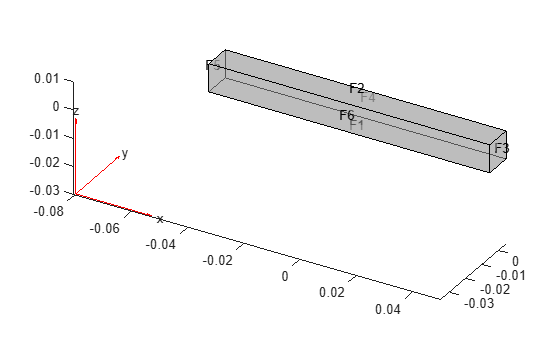# reduce

Reduce structural or thermal model

## Syntax

``Rcb = reduce(structuralmodel,"FrequencyRange",[omega1,omega2])``
``Rtherm = reduce(thermalmodel,"ModalResults",thermalModalR)``
``Rtherm = reduce(thermalmodel,"ModalResults",thermalModalR,"NumModes",N)``

## Description

example

````Rcb = reduce(structuralmodel,"FrequencyRange",[omega1,omega2])` reduces a structural analysis model to the fixed interface modes in the frequency range `[omega1,omega2]` and the boundary interface degrees of freedom.```

example

````Rtherm = reduce(thermalmodel,"ModalResults",thermalModalR)` reduces a thermal analysis model to the modes specified in `thermalModalR`. When reducing a thermal model, thermal properties of materials, internal heat sources, and boundary conditions cannot depend on time or temperature.```

example

````Rtherm = reduce(thermalmodel,"ModalResults",thermalModalR,"NumModes",N)` also truncates the number of modes to `N`. Using this syntax, you can compute a larger number of modes and then use a subset of these modes to construct a reduced-order model.```

## Examples

collapse all

Reduce a transient structural model to the fixed interface modes in a specified frequency range and the boundary interface degrees of freedom.

Create a transient structural model for a 3-D problem.

`structuralmodel = createpde("structural","transient-solid");`

Create a geometry and include it in the model. Plot the geometry.

```gm = multicuboid(0.1,0.01,0.01); structuralmodel.Geometry = gm; pdegplot(structuralmodel,"FaceLabels","on","FaceAlpha",0.5)```Specify Young's modulus, Poisson's ratio, and the mass density of the material.

```structuralProperties(structuralmodel,"YoungsModulus",70E9, ... "PoissonsRatio",0.3, ... "MassDensity",2700);```

Generate a mesh.

`generateMesh(structuralmodel);`

Specify the ends of the beam as structural superelement interfaces. The reduced-order model technique retains the degrees of freedom on the superelement interfaces while condensing the degrees of freedom on all other boundaries. For better performance, use the set of edges that bound each side of the beam instead of using the entire face.

```structuralSEInterface(structuralmodel,"Edge",[4,6,9,10]); structuralSEInterface(structuralmodel,"Edge",[2,8,11,12]);```

Reduce the model to the fixed interface modes in the frequency range `[-Inf,500000]` and the boundary interface degrees of freedom.

`R = reduce(structuralmodel,"FrequencyRange",[-Inf,500000])`
```R = ReducedStructuralModel with properties: K: [166x166 double] M: [166x166 double] NumModes: 22 RetainedDoF: [144x1 double] ReferenceLocations: [] Mesh: [1x1 FEMesh] ```

Reduce a thermal model using all modes or the specified number of modes from the modal solution.

Create a transient thermal model.

`thermalmodel = createpde("thermal","transient");`

Create a unit square geometry and include it in the model.

`geometryFromEdges(thermalmodel,@squareg);`

Plot the geometry, displaying edge labels.

```pdegplot(thermalmodel,"EdgeLabels","on") xlim([-1.1 1.1]) ylim([-1.1 1.1])```Specify the thermal conductivity, mass density, and specific heat of the material.

```thermalProperties(thermalmodel,"ThermalConductivity",400, ... "MassDensity",1300, ... "SpecificHeat",600);```

Set the temperature on the right edge to `100`.

`thermalBC(thermalmodel,"Edge",2,"Temperature",100);`

Set an initial value of `0` for the temperature.

`thermalIC(thermalmodel,0);`

Generate a mesh.

`generateMesh(thermalmodel);`

Solve the model for three different values of heat source and collect snapshots.

```tlist = 0:10:600; snapShotIDs = [1:10 59 60 61]; Tmatrix = []; heatVariation = [10000 15000 20000]; for q = heatVariation internalHeatSource(thermalmodel,q); results = solve(thermalmodel,tlist); Tmatrix = [Tmatrix,results.Temperature(:,snapShotIDs)]; end```

Switch the thermal model analysis type to modal.

`thermalmodel.AnalysisType = "modal";`

Compute the POD modes.

`RModal = solve(thermalmodel,"Snapshots",Tmatrix)`
```RModal = ModalThermalResults with properties: DecayRates: [6x1 double] ModeShapes: [1541x6 double] SnapshotsAverage: [1541x1 double] ModeType: "PODModes" Mesh: [1x1 FEMesh] ```

Reduce the thermal model using all modes in `RModal`.

`Rtherm = reduce(thermalmodel,"ModalResults",RModal) `
```Rtherm = ReducedThermalModel with properties: K: [7x7 double] M: [7x7 double] F: [7x1 double] InitialConditions: [7x1 double] Mesh: [1x1 FEMesh] ModeShapes: [1541x6 double] SnapshotsAverage: [1541x1 double] ```

Reduce the thermal model using only three modes.

```Rtherm3 = reduce(thermalmodel,"ModalResults",RModal, ... "NumModes",3)```
```Rtherm3 = ReducedThermalModel with properties: K: [4x4 double] M: [4x4 double] F: [4x1 double] InitialConditions: [4x1 double] Mesh: [1x1 FEMesh] ModeShapes: [1541x3 double] SnapshotsAverage: [1541x1 double] ```

## Input Arguments

collapse all

Structural model, specified as a `StructuralModel` object. The model contains the geometry, mesh, structural properties of the material, body loads, boundary loads, and boundary conditions.

Example: ```structuralmodel = createpde("structural","transient-solid")```

Frequency range, specified as a vector of two elements. Define `omega1` as slightly lower than the lowest mode's frequency and `omega2` as slightly higher than the highest mode's frequency. For example, if the lowest expected frequency is zero, then use a small negative value for `omega1`.

You can find natural frequencies and mode shapes for the specified frequency range by solving a modal analysis problem first. Then you can use a more precise frequency range to reduce the model. Note that a modal analysis problem still requires you to specify a frequency range. For example, see Modal Superposition Method for Structural Dynamics Problem.

Example: `[-0.1,1000]`

Data Types: `double`

Modal thermal analysis model, specified as a `ThermalModel` object. `ThermalModel` contains the geometry, mesh, thermal properties of the material, internal heat source, Stefan-Boltzmann constant, boundary conditions, and initial conditions.

Example: ```thermalmodel = createpde("thermal","modal")```

Modal analysis results for a thermal model, specified as a `ModalThermalResults` object.

Example: ```thermalModalR = solve(thermalmodel,"DecayRange",[0,1000])```

Number of modes, specified as a positive integer.

## Output Arguments

collapse all

Structural results obtained using the Craig-Bampton order reduction method, returned as a `ReducedStructuralModel` object.

Reduced-order thermal model, returned as a `ReducedThermalModel` object.

## Version History

Introduced in R2019b

expand all